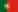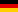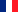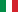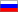### Blog

Before trying to explain what the GAUSS are, it is worth mentioning that the GAUSS is the measure of magnetic flux density but not necessarily the magnetic distance exerted by the body of the magnet to another body. For this need it is more reliable to measure it by the Kilo Grams of support that the magnet presents.

A gauss (G) is a unit of measurement of the magnetic field of the Cegesimal System of Units, named in honor of the German physicist-mathematician Karl Friedrich Gauss (1777-1855) who applied mathematical principles to a magnetic field.

The gauss is the unit with which the magnetic field is measured, it measures the magnetic flux density or also known as magnetic induction while the intensity of the magnetic field is measured by the oersted.

The Gauss rating of a magnet determines how fast it works, and the thickness determines the depth of penetration.

One gauss is defined as one maxwell per square centimeter.
1 gauss = 1 maxwell / cm2

The International System of Units unit of measurement for the magnetic field is the tesla.
10,000 gauss is equivalent to 1 tesla.
10,000 gauss = 1 tesla

Approximate GAUSS examples of…

• Human Brain: 0.000 0001 gauss (one millionth of a gauss)
• Earth's surface: 0.31 – 0.58 gauss
• Earth's core: 25 gauss
• Refrigerator magnet: 50 gauss
• Small iron magnet: 100 gauss
• Small neodymium magnet: 2,000 gauss (0.2 tesla)
• Electrified magnet or electro-magnet: 15,000 gauss (1.5 tesla)
• Medical magnetic resonance machine: 70,000 gauss (7 tesla)
• Strong laboratory magnet: 100,000 gauss (10 tesla)
• Star Magnetar: 1,000,000,000,000,000 gauss (one thousand trillion gauss or 1 trillion tesla (large scale figures))
• Neutron star: 100,000,000,000,000,000 gauss (one hundred thousand trillion gauss or 10 trillion telsas (large scale figures)):

The latter is the upper limit to the magnetism studied, there is no magnetic field stronger than that known in the universe.

How to know how many Gauss has a magnet.

A magnet emits 2 different GAUSS measurements.

EXTERNAL GAUSS
INTERNAL GAUSS

Some web pages and companies that sell magnets put the Gauss number without mentioning if they are internal or external, for which they put the highest figure in this way without mentioning the external Gauss number.
Many manufacturers of magnetic products do not specify the Gauss number on their products due to the confusion that the rating can cause.

EXTERNAL GAUSS:

With a GAUSIMETER it is possible to know automatically and exactly how many external GAUSS emits a MAGNET, the GAUSS number can also be calculated by means of a complex mathematical equation of Maxwell.

INTERNAL GAUSS:

To know the approximate number of internal gauss, 3.95 must be multiplied by the number of external gauss, but if the resulting number exceeds 12,800 gauss, it will no longer be possible to trust this result, since this is normally the maximum gauss. that can contain a Neodymium or Alnico magnet. The minimum internal force has to be 3,950.

For example.

A 1,000 external gauss magnet will contain very approximately 3,950 internal gauss.

The Gaussian rating of a magnet can be misleading, as the magnetic field strength falls off the surface of the magnet very quickly as it moves away from the target zone.

The magnet by itself does not determine its strength or magnetic flux or total gauss and for this they are considered.

• The material (Alnico, Smco, NdFeB, Ferrite)
• The diameter (determines the width)
• The thickness (determines the flow force)
• The degree of magnetization.

What penetration depth towards objects or the body do the magnets have with respect to the amount of gauss it contains?

• Larger magnets create wider but not more powerful fields.
• It is the thickness that determines the power after the degree of magnetization.
• The wider the field, the lower the power.
• A magnet has to be strong enough to penetrate deep into human tissue.

For example, to penetrate deeply it must be…

• A Ferrite magnet must have at least 3,850 internal gauss equivalent to 987 external gauss.
• A medium magnetization neodymium magnet easily manages to exceed this minimum so it can reach deeper tissues and to penetrate…
• 1 to 3" inch which must have a minimum 987 gauss external strength rating.
• 3 to 6" inches of penetration the magnet shall have a 2,800 to 3,200 gauss external rating.

Research and writing by:

JOE PARDO
I-MANES® / skuers®
Founder
i n f o @ i - m a n e s . com

IMANES® 2013 - 2015

*The information written in this article is from the I-MANES.com team's own research to inform the community in the most accurate way on the subject, we are working to investigate, experiment and verify more of this information.